In :
%matplotlib inline
%config InlineBackend.figure_format = 'retina'

import numpy as np
import pandas as pd
from lifelines.fitters.piecewise_exponential_regression_fitter import PiecewiseExponentialRegressionFitter
from lifelines import *
from lifelines.generate_datasets import piecewise_exponential_survival_data

In :
# this code can be skipped
# generating piecewise exponential data to look like a monthly churn curve.

N, d = 2000, 2

# some numbers take from http://statwonk.com/parametric-survival.html
breakpoints = (1, 31, 34, 62, 65, 93, 96)

betas = np.array(
[
[1.0, -0.2, np.log(15)],
[5.0, -0.4, np.log(333)],
[9.0, -0.6, np.log(18)],
[5.0, -0.8, np.log(500)],
[2.0, -1.0, np.log(20)],
[1.0, -1.2, np.log(500)],
[1.0, -1.4, np.log(20)],
[1.0, -3.6, np.log(250)],
]
)

X = 0.1 * np.random.exponential(size=(N, d))
X = np.c_[X, np.ones(N)]

T = np.empty(N)
for i in range(N):
lambdas = np.exp(-betas.dot(X[i, :]))
T[i] = piecewise_exponential_survival_data(1, breakpoints, lambdas)

T_censor = np.minimum(T.mean() * np.random.exponential(size=N), 110) # 110 is the end of observation, eg. current time.

df = pd.DataFrame(X[:, :-1], columns=['var1', 'var2'])
df["T"] = np.minimum(T, T_censor)
df["E"] = T <= T_censor

In :
df.head()

Out:
var1 var2 T E
0 0.302575 0.170184 68.502873 False
1 0.094912 0.046740 110.000000 False
2 0.112535 0.052899 52.589615 False
3 0.125926 0.016203 10.558145 True
4 0.088505 0.080449 110.000000 False
In :
kmf = KaplanMeierFitter().fit(df['T'], df['E'])
kmf.plot(figsize=(11,6))

Out:
<matplotlib.axes._subplots.AxesSubplot at 0x11fdcacf8>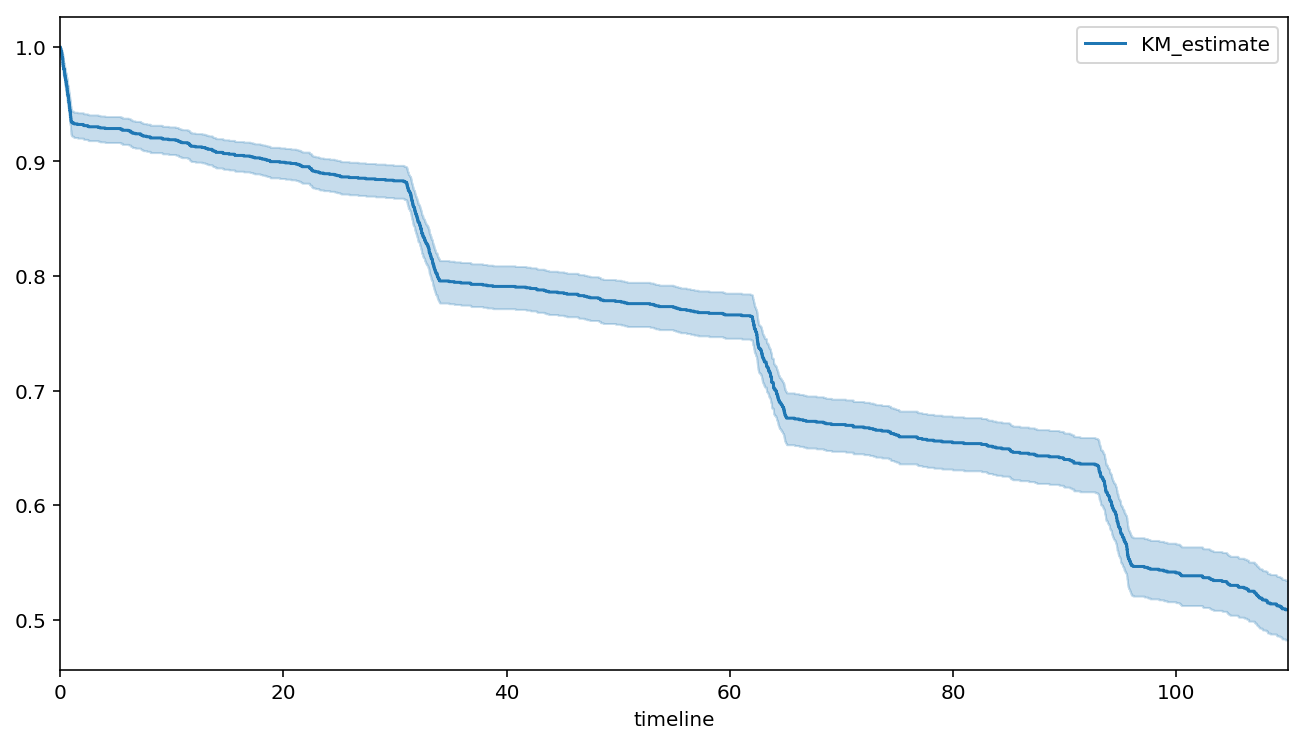To borrow a term from finance, we clearly have different regimes that a customer goes through: periods of low churn and periods of high churn, both of which are predictable.

Let's fit a piecewise hazard model to this curve. Since we have baseline information, we can fit a regression model. For simplicity, let's assume that a customer's hazard is constant in each period, however it varies over each customer (heterogenity in customers).

Our hazard model looks like¹: $$h(t\;|\;x) = \begin{cases} \lambda_0(x)^{-1}, & t \le \tau_0 \\ \lambda_1(x)^{-1} & \tau_0 < t \le \tau_1 \\ \lambda_2(x)^{-1} & \tau_1 < t \le \tau_2 \\ ... \end{cases}$$

and $\lambda_i(x) = \exp(\mathbf{\beta}_i x^T), \;\; \mathbf{\beta}_i = (\beta_{i,1}, \beta_{i,2}, ...)$. That is, each period has a hazard rate, $\lambda_i$ the is the exponential of a linear model. The parameters of each linear model are unique to that period - different periods have different parameters (later we will generalize this).

Why do I want a model like this? Well, it offers lots of flexibilty (at the cost of efficiency though), but importantly I can see:

1. Influence of variables over time.
2. Looking at important variablas at specific "drops" (or regime changes). For example, what variables cause the large drop at the start? What variables prevent death at the second billing?
3. Predictive power: since we model the hazard more accurately (we hope) than a simpler parametric form, we have better estimates of a subjects survival curve.

¹ I specifiy the reciprocal because that follows lifelines convention for exponential and weibull hazards. In practice, it means the interpretation of the sign is possibly different.

In :
pew = PiecewiseExponentialRegressionFitter(
breakpoints=breakpoints)\
.fit(df, "T", "E")

pew.print_summary()

<lifelines.PiecewiseExponentialRegressionFitter: fitted with 2000 observations, 1234 censored>
duration col = 'T'
event col = 'E'
number of subjects = 2000
number of events = 766
log-likelihood = -3823.68
time fit was run = 2019-03-27 18:49:23 UTC

---
coef exp(coef)  se(coef)     z      p  -log2(p)  lower 0.95  upper 0.95
lambda_0_ var1        0.04      1.04      0.90  0.05   0.96      0.06       -1.72        1.81
var2       -0.76      0.47      0.88 -0.87   0.39      1.37       -2.48        0.96
_intercept  2.76     15.86      0.15 18.13 <0.005    241.61        2.47        3.06
lambda_1_ var1        6.02    413.39      1.65  3.65 <0.005     11.87        2.79        9.26
var2       -0.60      0.55      1.04 -0.58   0.56      0.82       -2.64        1.44
_intercept  5.85    347.13      0.18 32.85 <0.005    783.84        5.50        6.20
lambda_2_ var1        7.03   1133.55      1.40  5.03 <0.005     20.97        4.29        9.77
var2       -0.60      0.55      0.85 -0.71   0.48      1.07       -2.27        1.06
_intercept  2.90     18.11      0.15 19.90 <0.005    290.29        2.61        3.18
lambda_3_ var1        4.25     70.43      2.02  2.11   0.03      4.84        0.30        8.21
var2       -3.73      0.02      1.20 -3.11 <0.005      9.07       -6.08       -1.38
_intercept  6.62    753.41      0.26 25.55 <0.005    475.77        6.12        7.13
lambda_4_ var1        2.90     18.11      1.14  2.54   0.01      6.49        0.66        5.13
var2       -2.00      0.14      0.88 -2.28   0.02      5.47       -3.71       -0.28
_intercept  3.14     23.19      0.16 19.81 <0.005    287.86        2.83        3.45
lambda_5_ var1        3.84     46.72      1.77  2.17   0.03      5.05        0.37        7.32
var2       -2.33      0.10      1.26 -1.86   0.06      3.99       -4.80        0.13
_intercept  5.95    384.10      0.24 25.31 <0.005    467.08        5.49        6.41
lambda_6_ var1        2.60     13.46      1.13  2.29   0.02      5.52        0.38        4.82
var2       -3.95      0.02      0.83 -4.76 <0.005     18.95       -5.57       -2.32
_intercept  3.18     23.98      0.17 18.61 <0.005    254.41        2.84        3.51
lambda_7_ var1       -0.15      0.86      1.35 -0.11   0.91      0.14       -2.79        2.49
var2       -2.23      0.11      1.61 -1.38   0.17      2.59       -5.39        0.93
_intercept  5.49    243.11      0.26 21.06 <0.005    324.81        4.98        6.00
---
Concordance = 0.57
Log-likelihood ratio test = 115.61 on 22 df, -log2(p)=46.39

In :
fig, ax = plt.subplots(figsize=(10, 10))
pew.plot(ax=ax)

Out:
<matplotlib.axes._subplots.AxesSubplot at 0x122947ba8>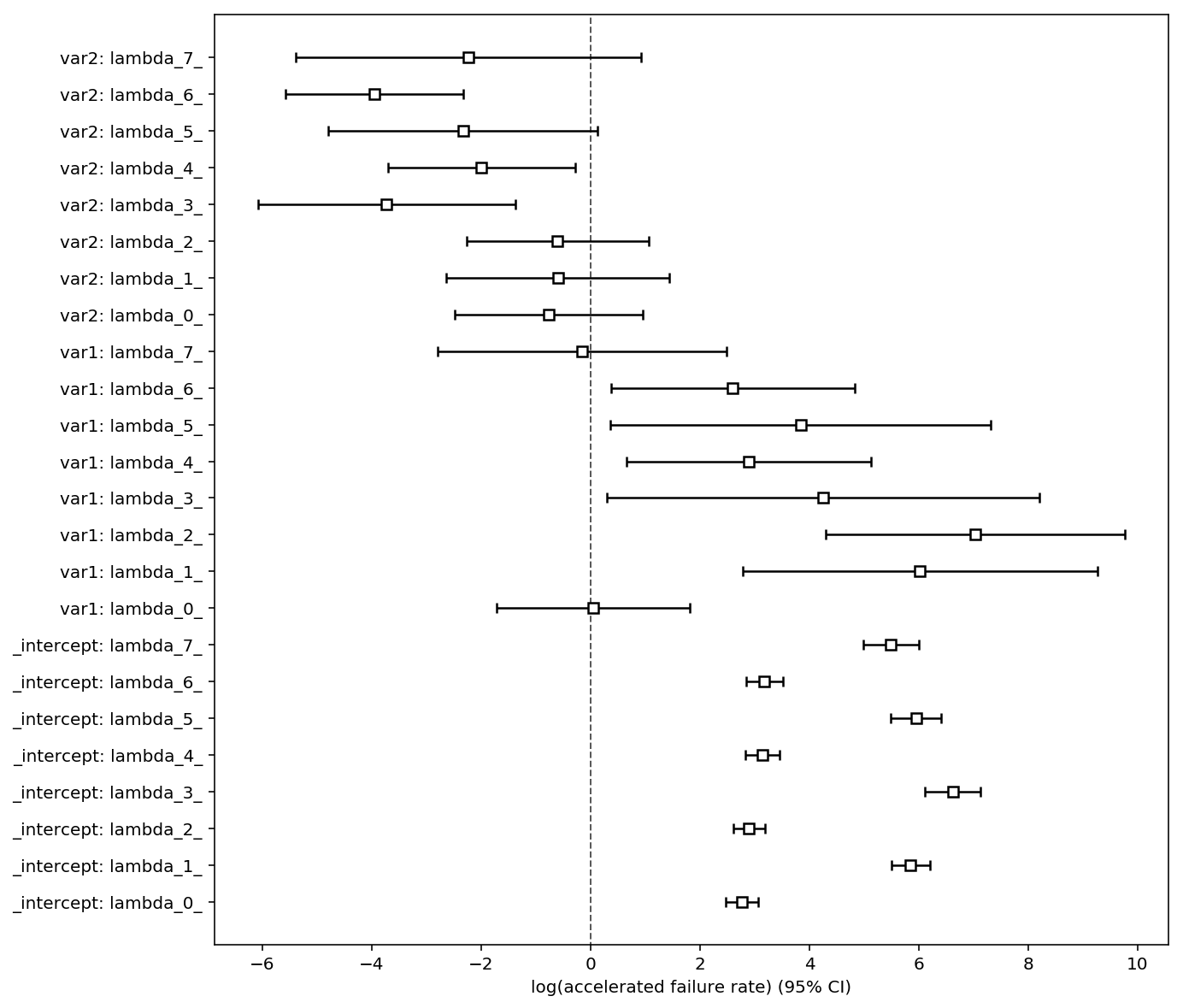If we suspect there is some parameter sharing between pieces, or we want to regularize (and hence share information) between pieces, we can include a penalizer which penalizes the variance of the estimates per covariate.

Specifically, our penalized log-likelihood, $PLL$, looks like:

$$PLL = LL - \alpha \sum_j \hat{\sigma}_j^2$$

where $\hat{\sigma}_j$ is the standard deviation of $\beta_{i, j}$ over all periods $i$. This acts as a regularizer and much like a multilevel component in Bayesian statistics.

Below we examine some cases of $\alpha$.

Note: we do not penalize the intercept, currently. This is a modellers decision, but I think it's better not too.

In :
# Extreme case, note that all the covariates' parameters are almost identical.
pew = PiecewiseExponentialRegressionFitter(
breakpoints=breakpoints,
penalizer=20.0)\
.fit(df, "T", "E")

fig, ax = plt.subplots(figsize=(10, 10))
pew.plot(ax=ax)

Out:
<matplotlib.axes._subplots.AxesSubplot at 0x121e8f160>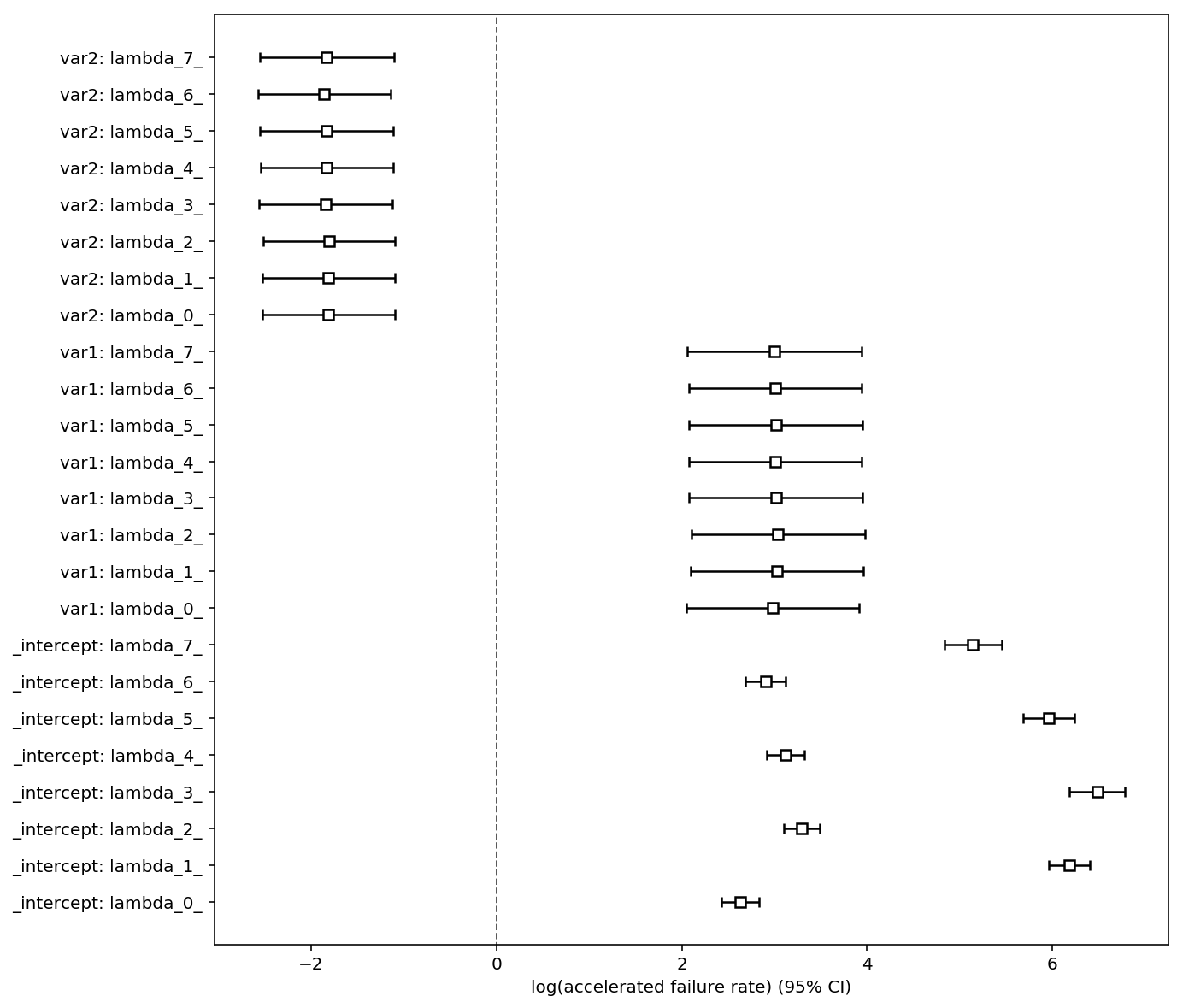In :
# less extreme case
pew = PiecewiseExponentialRegressionFitter(
breakpoints=breakpoints,
penalizer=.25)\
.fit(df, "T", "E", timeline=np.linspace(0, 130, 200))

fig, ax = plt.subplots(figsize=(10, 10))
pew.plot(ax=ax, fmt="s", label="small penalty on variance")

# compare this to the no penalizer case

pew_no_penalty = PiecewiseExponentialRegressionFitter(
breakpoints=breakpoints,
penalizer=0)\
.fit(df, "T", "E", timeline=np.linspace(0, 130, 200))

pew_no_penalty.plot(ax=ax, c="r", fmt="o", label="no penalty on variance")
plt.legend()

Out:
<matplotlib.legend.Legend at 0x123a5eac8>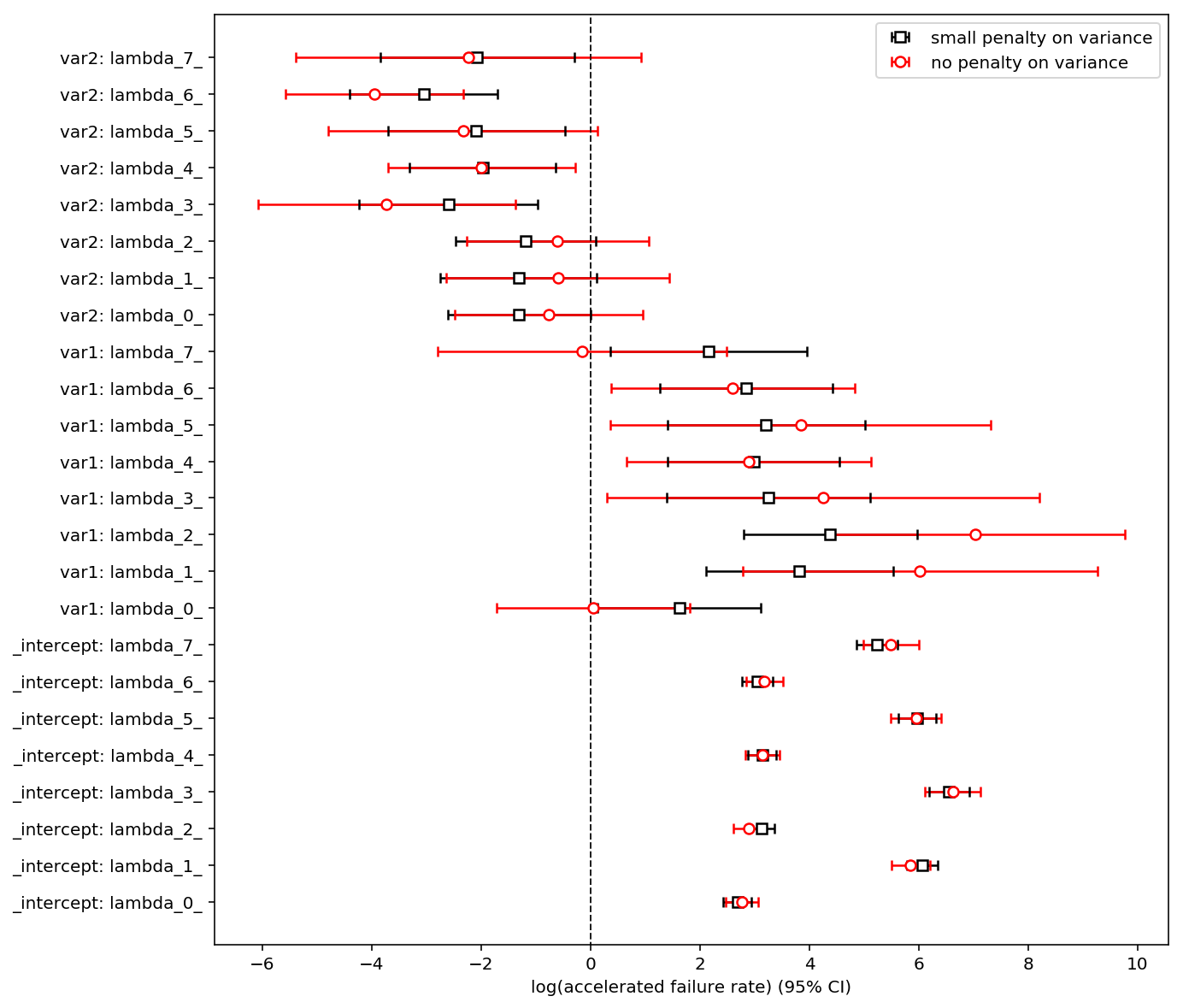In :
# Some prediction methods

pew.predict_survival_function(df.loc[0:5]).plot(figsize=(10, 5))

Out:
<matplotlib.axes._subplots.AxesSubplot at 0x124198080>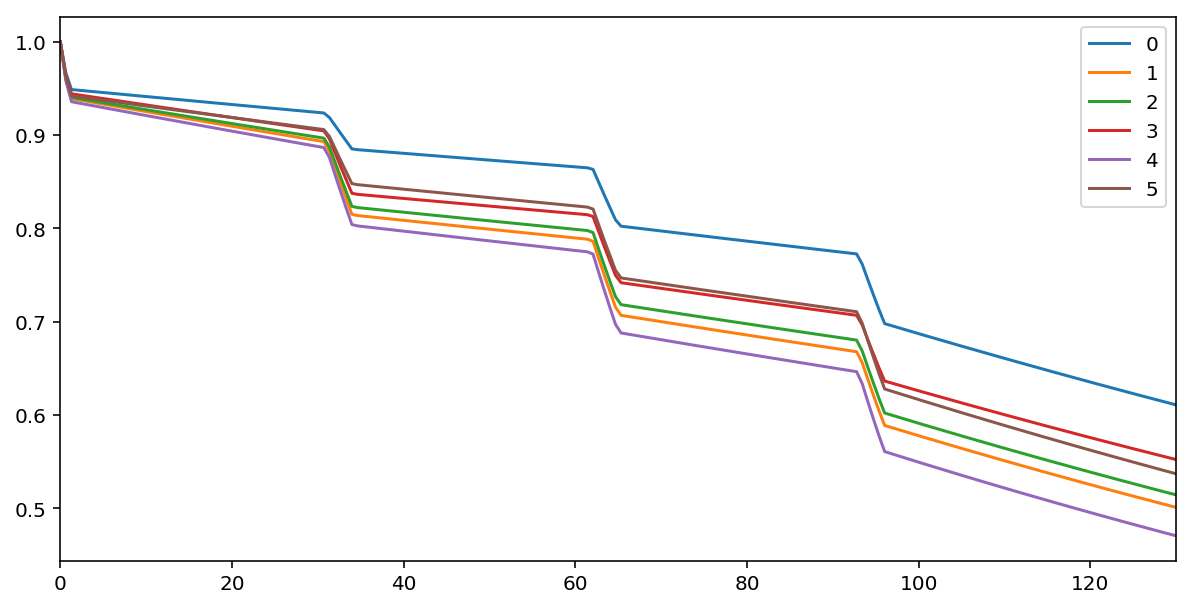In :
pew.predict_cumulative_hazard(df.loc[0:5]).plot(figsize=(10, 5))

Out:
<matplotlib.axes._subplots.AxesSubplot at 0x1241a18d0>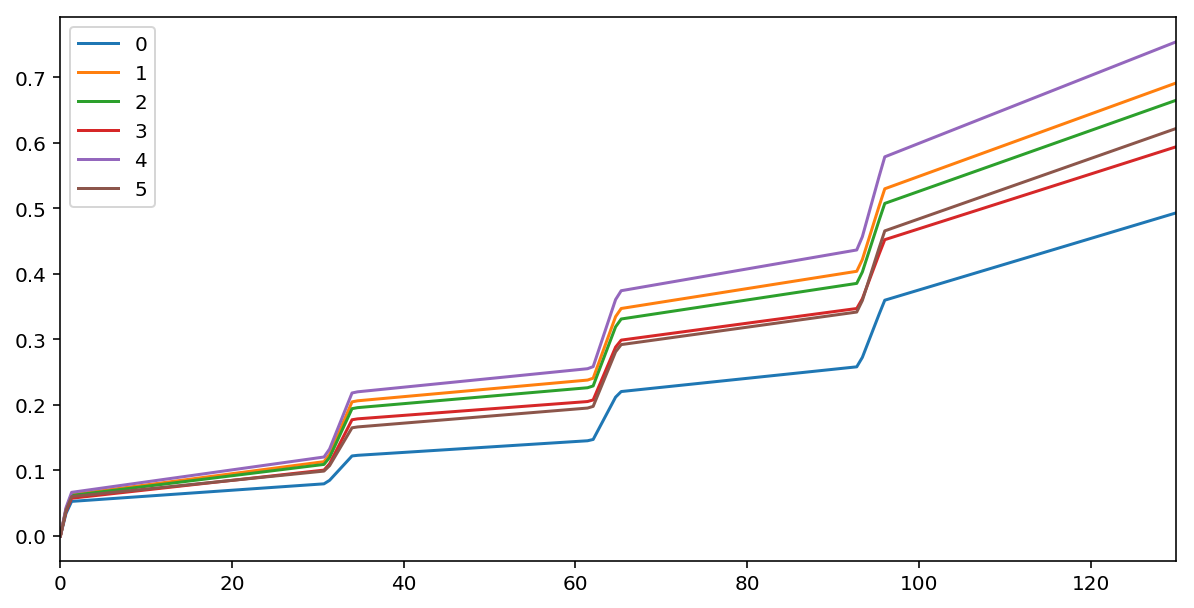In :
pew.predict_median(df.loc[0:5])

Out:
0.5
0 inf
1 inf
2 inf
3 inf
4 118.241206
5 inf
In :
# hazard
pew.predict_cumulative_hazard(df.loc[0:5]).diff().plot(figsize=(10, 5))

Out:
<matplotlib.axes._subplots.AxesSubplot at 0x11fd0beb8>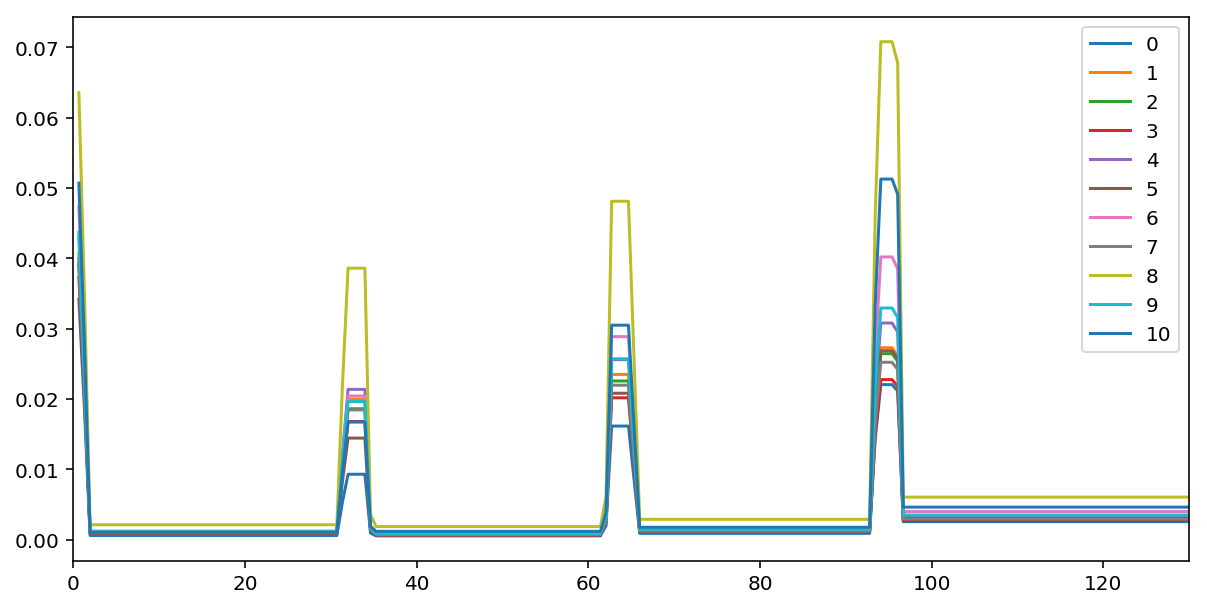In [ ]:


In :
pew.print_summary()

<lifelines.PiecewiseExponentialRegressionFitter: fitted with 2000 observations, 1234 censored>
duration col = 'T'
event col = 'E'
penalizer = 0.25
number of subjects = 2000
number of events = 766
log-likelihood = -3836.07
time fit was run = 2019-03-27 18:49:26 UTC

---
coef exp(coef)  se(coef)     z      p  -log2(p)  lower 0.95  upper 0.95
lambda_0_ var1        1.62      5.06      0.76  2.13   0.03      4.90        0.13        3.12
var2       -1.31      0.27      0.67 -1.96   0.05      4.31       -2.62        0.00
_intercept  2.68     14.60      0.13 20.62 <0.005    311.43        2.43        2.94
lambda_1_ var1        3.82     45.57      0.87  4.37 <0.005     16.29        2.11        5.53
var2       -1.32      0.27      0.73 -1.81   0.07      3.82       -2.75        0.11
_intercept  6.07    432.40      0.14 42.63 <0.005       inf        5.79        6.35
lambda_2_ var1        4.38     80.10      0.81  5.41 <0.005     23.89        2.79        5.97
var2       -1.19      0.30      0.65 -1.82   0.07      3.88       -2.47        0.09
_intercept  3.13     22.83      0.12 25.66 <0.005    479.96        2.89        3.37
lambda_3_ var1        3.25     25.70      0.95  3.42 <0.005     10.65        1.39        5.11
var2       -2.60      0.07      0.83 -3.12 <0.005      9.12       -4.23       -0.97
_intercept  6.55    702.63      0.19 34.59 <0.005    868.74        6.18        6.93
lambda_4_ var1        2.98     19.65      0.80  3.73 <0.005     12.33        1.41        4.54
var2       -1.98      0.14      0.68 -2.89 <0.005      8.02       -3.32       -0.64
_intercept  3.14     22.99      0.13 23.70 <0.005    410.12        2.88        3.39
lambda_5_ var1        3.21     24.75      0.92  3.48 <0.005     10.94        1.40        5.02
var2       -2.09      0.12      0.83 -2.52   0.01      6.42       -3.71       -0.46
_intercept  5.97    392.92      0.18 33.95 <0.005    836.83        5.63        6.32
lambda_6_ var1        2.84     17.16      0.80  3.54 <0.005     11.28        1.27        4.42
var2       -3.05      0.05      0.69 -4.42 <0.005     16.61       -4.41       -1.70
_intercept  3.05     21.09      0.14 21.56 <0.005    339.96        2.77        3.33
lambda_7_ var1        2.15      8.63      0.92  2.35   0.02      5.72        0.35        3.96
var2       -2.07      0.13      0.91 -2.29   0.02      5.49       -3.85       -0.29
_intercept  5.24    188.72      0.19 27.49 <0.005    550.11        4.87        5.61
---
Concordance = 0.57
Log-likelihood ratio test = 90.83 on 22 df, -log2(p)=31.91

In :
from lifelines import WeibullAFTFitter
wf = WeibullAFTFitter().fit(df, 'T', 'E')

In [ ]: